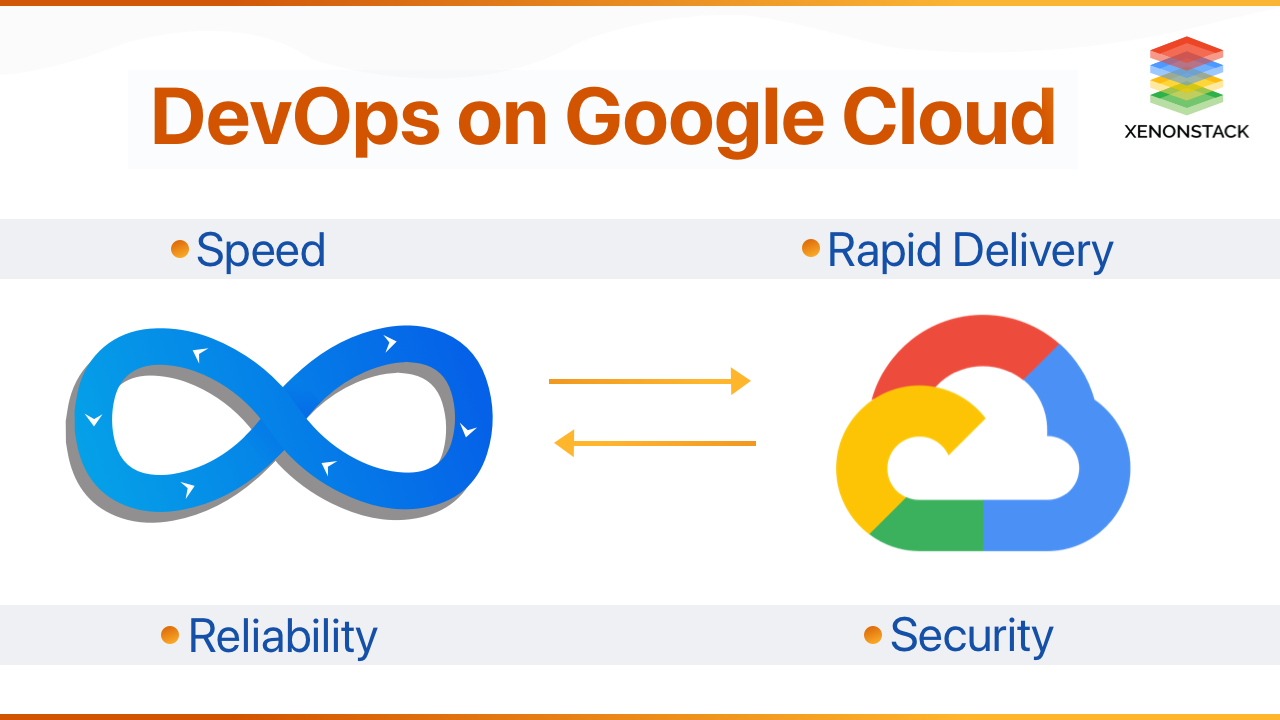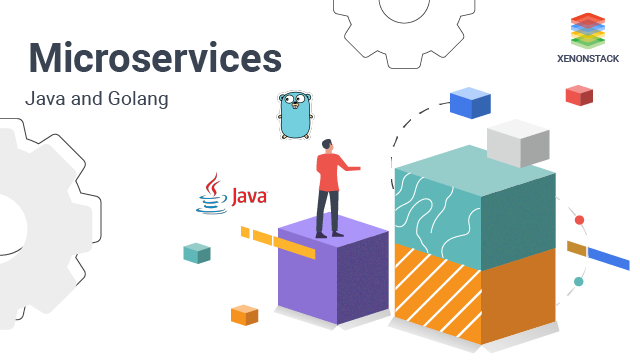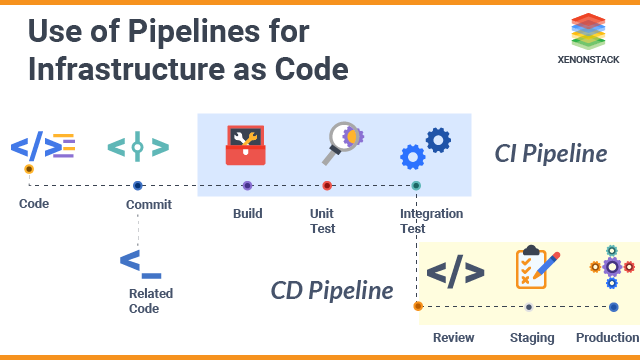#### XenonStack Recommends

Deployment Automation

# Probability Forecasting Benefits and its Use Cases | Quick Guide

Gursimran Singh | 26 May 2023

## Introduction to Probability Forecasting

In probability forecasting, we summarize what we know about or believe about the future. Forecasting probabilities includes expressing outcomes or events as quantities in numerical format. Probabilistic forecasts assign certainty to various outcomes, whereas single-valued forecasts assign only one outcome to each probability. Thus probability forecasting can be regarded as probability output based on class inputs.

As the word suggests, probability forecasting is summarized by two words

Probability and Forecasting: Probability is defined as the likeness of any event (i.e., how likely the event can occur), and Forecasting is the prediction of the occurrence of something with the help and reference of a similar previous event. A probabilistic forecast contains a set of probabilities associated with all possible future outcomes instead of just one particular outcome being pinpointed across the forecast.

### Why Probabilistic Forecasting is essential?

whenever there is a certain level of uncertainty in a system that might not be reduced, which is mostly the case when complex systems are involved. Forecasts based on probabilistic models are essential to ensure robust decisions are made during supply chain operations. Probabilistic Forecasting provides an optimal way to address demand and lead time, two critical factors in a supply chain analysis. A probabilistic viewpoint lends itself perfectly well to the economic prioritization of a decision based on its expected but uncertain returns since this perspective is based on the probability of the outcomes. It is possible to make probabilistic forecasts using various statistical models. Some are structurally similar to their deterministic counterparts, while others differ in their structural approach. Several metrics must be measured to judge the accuracy of a probabilistic forecast, as opposed.

To one based on deterministic data. To exploit probabilistic forecasts in their entirety, special tools must be developed that differ from those used to analyze deterministic forecasts.

The use of data and analytics techniques to improve the performance of the supply chain. Taken From Article, Supply Chain Analytics and its Use Cases

## Why do Supply Chains need Probability Forecasting?

A downside to having multiple forecasting methods is that each is designed for a different type of scenario. A probabilistic demand planning system works similarly to the human mind as opposed to algorithms designed a century ago. It is needed as it helps organizations and industries understand outcomes by utilizing data and the product's performance.

Probabilistic Forecasting is a vital tool for supply chain management because it helps companies to make informed decisions about their operations and mitigate risks. The supply chain is often subject to various sources of uncertainty, such as changes in demand, supply disruptions, and natural disasters. Probabilistic Forecasting helps companies to quantify and account for this uncertainty, allowing them to make better-informed decisions.

## What are the various Steps in Probability Forecasting?

The steps carried out in probability forecasting may vary per the algorithm considered for probability. But in general, these steps are used in probability forecasting:

• Purpose-based Data Collection: One must first determine what one wants to forecast and then gather relevant data that can be used for training the forecast and developing the probability.
• Data Processing: Data needs to be cleaned and prepared for analysis by eliminating missing values, outliers, and plagiarism and transforming the data to make it more accurate.
• Selection of Model: Determine which algorithm best suits the problem statement based on the data and expected accuracy. The model may also be selected based on a trend that was successful in the past analysis as per data valuation.
• Model Fitting: Training the model on the data to estimate its parameters. It can be tested with data to determine how the model might perform upon application.
For example, Dry Run.
• Evaluation of Model: It is essential to compare predictions made by the model to actual results to evaluate its performance. It also explains how the probability model will last in the long run.
• Model Improvement: Continually improving a model by adjusting its parameters, using a different algorithm, or gathering additional data with each iteration or repetition.
• Predictions: The trained model can make predictions by frequently inputting updated data into the model to keep up with current events.
• Post-processing: The predictions should be presented meaningfully by explaining them and elaborately. There is a lot of information to consider when in the process of probability forecasting, and to achieve the desired level of accuracy desired; it is widespread to repeat steps 4-7 multiple times to get the desired results.

## Algorithms of Probability Forecasting

The probability forecasting technique uses mathematical models and statistical methods to predict what will happen in the future. Different algorithms are used in probability forecasting depending on the problem and the data.

• Time Series Analysis: This model is based on a series of data points over time, which can be used to predict what the future will bring. It takes input according to the time and analyzes them to predict the output of data for the future based on present monitoring and bandwidth from past
• Regression Analysis: In this process, independent variables are used to predict the values of a dependent variable by using a relationship between them. The dependent values are compared in parallel to independent values to get the trend in which the values stand out or evaluate in the long run.
• Bayesian Analysis: This algorithmic analysis works on the principle of Bayes' theorem to predict upon obtaining the probabilities of random events based on new and unique information. According to Bayes' Theorem, the likelihood of a given event occurring based on the probability of another event occurring is equal to the likelihood of both events occurring given the first event has occurred multiplied by the probability of the first event taking place. For example, Bayes' theorem allows a more accurate assessment of health risks for individuals of known ages by conditioning the risk about their age instead of simply assuming that the individual is typical of the population.
• Machine learning Algorithms: For making predictions, various algorithms are available, such as decision trees, random forests, support vector machines, and neural networks. Machine learning analysis uses ML (Machine Learning)techniques and models to provide results for any data analysis. For example, AWS (Amazon Web Services) Sage Maker service is used for creating machine learning models using data as input and deploying those models for further application.
• Exponential Smoothing: This approach aims to smooth out time series data by assigning higher weights to recent observations and lower weights to older observations. These time series data can further be used along with Machine learning models for better data probability insight as it is already separated as per weights. To determine which algorithm will work best, a variety of factors should be considered, such as how much data are available, the type of data, the complexity of the problem, and the desired level of accuracy. To choose the most effective algorithm for a given issue, one should understand the assumptions and limitations of each algorithm.
Helping enterprises to cost savings, greater predictability, and improved availability of the systems. Taken From Article, Predictive Maintenance using Machine learning

## What are the major benefits of Probability Forecasting?

It is common for demand forecasts to be partial or incomplete: Only one number is provided: the most likely value of future demand. This is often regarded as a point forecast. In general, point forecasts estimate future demand on average. An estimate of future demand is much more helpful if it is based on a full probability distribution. Probability forecasting is more commonly used and valuable than scenario planning.

### Probability of Accurate Result

A point forecast only provides a partial view of the future, such as (i.e., the most likely demand in the upcoming time). In contrast, a probability forecast provides even the proper insight and the outcome of demand ( i.e., how much is the risk of demand shortage). By collecting this information, you can evaluate risks and prepare contingency plans. Because most point forecasts have only a tiny probability of accuracy, future planning of such events is necessary.
For example, there is also a possibility that a probability forecast will say something like: "Even though 15 units is the most likely value for demand, there is only a 10% chance that the forecast will be wrong. Hence, the point forecast has a 90% chance of being incorrect. "Forecasting errors of this kind are not a mistake: they are part of dealing with dynamic demand. These types of errors can often be regarded as uncertainty

### Application of Inventory Management

To manage inventory effectively, you must balance the availability of items with the inventory cost. To set reorder points (mins) on a rational, scientific basis, knowing the full probability distribution of demand during a replenishment lead time is necessary.

### High Insight into Data

A probability forecast provides extra information that can make a significant difference in probability forecasting, so it is always better to know more than to know less. The ability of software to produce point forecasts has been available for a long time; however, modern software is capable of much more and can provide a much more thorough picture with the application of probability forecasting.

### Enhanced Competitiveness

Probability forecasting is one of the best ways for organizations to make data-driven decisions, gaining a competitive advantage over those who use intuition and guesswork to make their decisions.

An organization can identify and mitigate potential risks by using probability forecasts, reducing the likelihood of adverse events, and increasing the probability of success. Probability forecasting can provide organizations with the possibility of allocating resources more efficiently and minimizing the wastage of these resources, thus freeing up the inventory. The resources are so freed up that they can be deployed in other areas where they can be used better.

## Use Cases of Probability Forecasting

An effective way to optimize a supply chain is to make the "right" decision each time that is made - at the moment - that is most likely to make a profit in addressing a future situation left to be estimated with only a little accuracy. Despite this, to a great extent, a high degree of uncertainty is associated with future events. This can further be reduced using probabilistic Forecasting as a prediction of event occurrence in the supply chain can help to reduce problems and errors that might occur otherwise.
With a probabilistic forecasting approach, we take a radical or fundamental view of uncertainty in the supply chain. To put it another way, we strive to define and quantify the uncertainty in as detail as possible, using a comprehensive assessment of all possible factors. Many aspects of supply chains are particularly suitable for probabilistic Forecastings, such as:

• Demand: Various products, such as garments, accessories, and spare parts, tend to have erratic or intermittent demand. There are no guarantees when it comes to product launches. There is always the possibility of large portions of market shares being replaced and destroyed by its competitors on a temporary and irregular basis using marketing and promotions.
• Yield: In the case of fresh food, yield and quality are determined by factors beyond the company's control, such as the weather. Forecasts based on probabilities quantify these factors for the entire season, allowing for more accurate predictions than classic forecasts.
• Returns: The odds of two of the three sizes being returned are high when a customer orders the same product in three different sizes. Although regional differences exist in return policies, customers tend to take advantage of them when available. Each order should be evaluated for its probability of returning which may help in forecasting the financial loss and analyzing to avoid any such event.
• Lead Time: The lead time for overseas imports can vary greatly depending on where they are produced, shipped, and received. If there is a stock-out problem, local suppliers may experience longer lead times.Processing images is deep, labour intense work along a data-centric process, Computer vision requires deep learning models. Computer Vision Services and Solutions

## Conclusion

The purpose of probability forecasts is not to be able to predict an atmospheric variable precisely for an upcoming period. Instead of predicting a variable's future movement, probability forecasts describe how likely it is to fall into a defined range. In short, probability forecasts provide proper signals only over long periods, which makes them ideal for long-range Forecasting. In addition to conveying the output of a group of similar forecasts, probability forecasts are also helpful in conveying ensemble forecasts. Probability forecasts can judge the outcome on their own; their success or reliability can only be determined if there is a significant number of similar forecasts, and their results can be evaluated collectively to generate the exact Forecasting of demand.

Probabilistic Forecasting is a valuable tool for supply chain management because it helps companies make more informed decisions and reduce risks in the face of uncertainty.

## Related InsightsDeployment Automation

### DevOps on Google Cloud Platform - Complete GuideDeployment Automation

### Microservices for Java and Golang - A Complete SolutionDeployment Automation